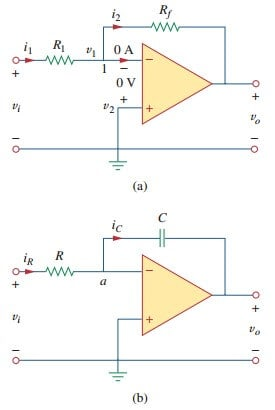# Op Amp Integrator Circuit and ExampleThis op amp integrator circuit is one of the implementation between op amp and capacitor. Important op amp circuits that use energy-storage elements include integrators and differentiators. These op amp circuits often involve resistors and capacitors; inductors (coils) tend to be bulkier and expensive.

## Op Amp Integrator Circuit

The op amp integrator is used in numerous applications, especially in analog computers.

An integrator is an op amp circuit whose output is proportional to the integral of the input signal.

If the feedback resistor Rf in the familiar inverting amplifier of Figure.(1a) is replaced by a capacitor, we obtain an ideal integrator, as shown in Figure.(1b).Figure 1. Replacing the feedback resistor in the inverting amplifier in (a) produces an integrator in (b).

It is interesting that we can obtain a mathematical representation of integration this way. At node a in Figure.(1b),

ButSubstituting these in Equation.(1), we obtain

Integrating both sides gives

To ensure that vo(0) = 0, it is always necessary to discharge the integrator’s capacitor prior to the application of a signal. Assuming vo(0) = 0,

which shows that the circuit in Figure.(1b) provides an output voltage proportional to the integral of the input. In practice, the op amp integrator requires a feedback resistor to reduce dc gain and prevent saturation.

Care must be taken that the op amp operates within the linear range so that it does not saturate.

## Op Amp Integrator Example

If v1 = 10 cos 2 t mV and v2 = 0.5 t mV, find vo in the op amp circuit in Figure.(2). Assume that the voltage across the capacitor is initially zero.